Miscellaneous

Chapter 9 Class 11 Sequences and Series
Serial order wise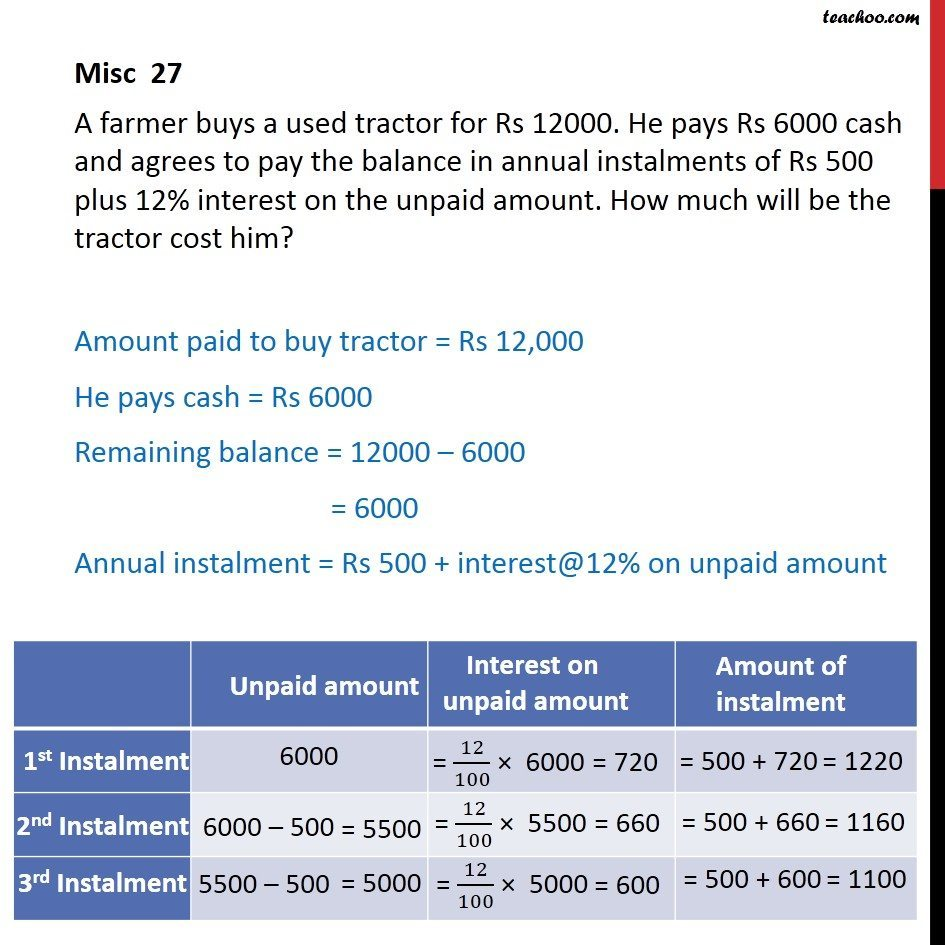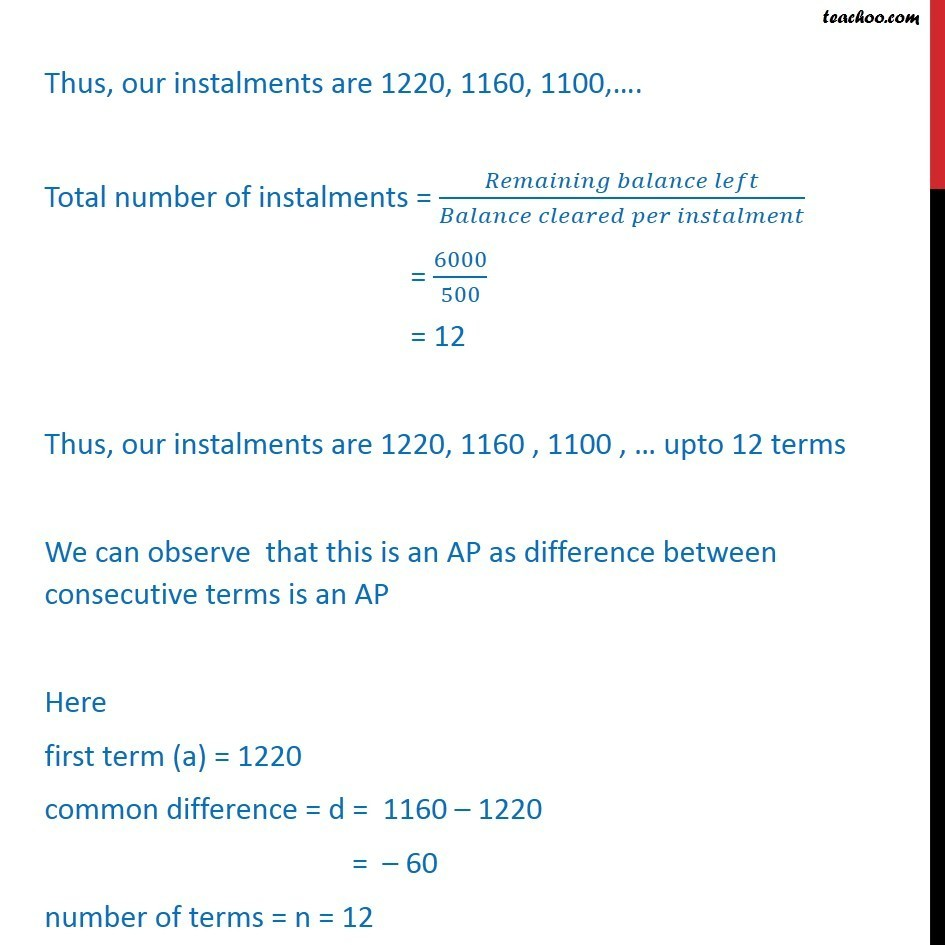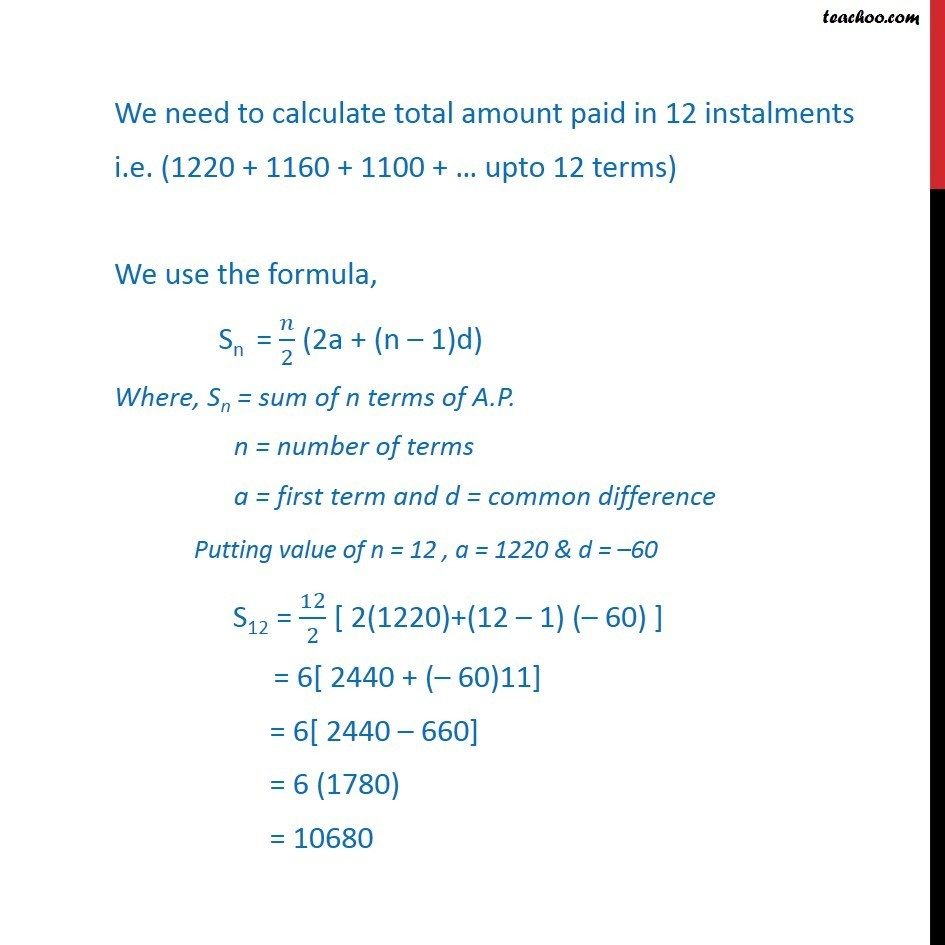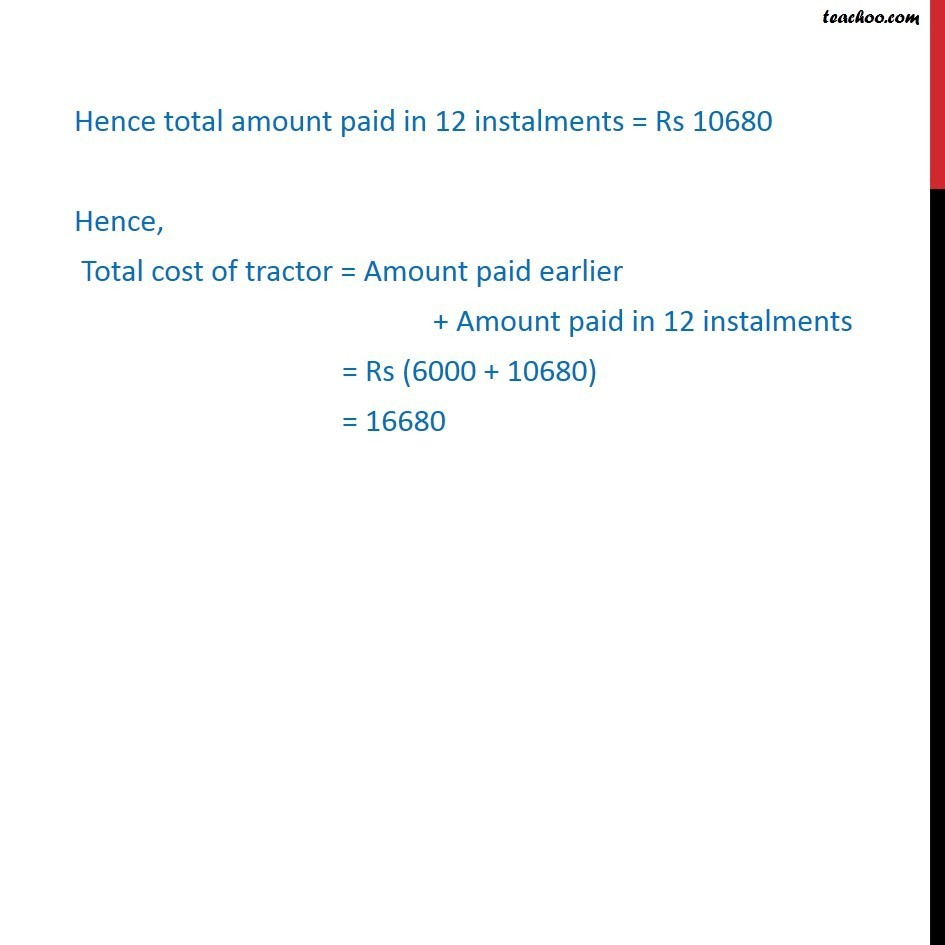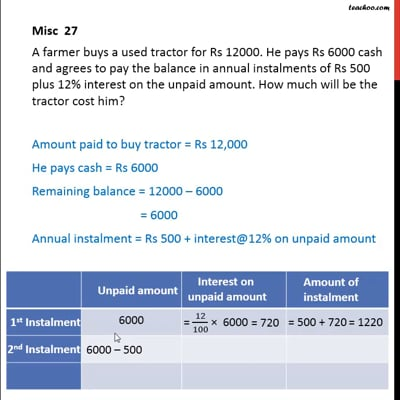This video is only available for Teachoo black users

Introducing your new favourite teacher - Teachoo Black, at only ₹83 per month

### Transcript

Misc 27 A farmer buys a used tractor for Rs 12000. He pays Rs 6000 cash and agrees to pay the balance in annual instalments of Rs 500 plus 12% interest on the unpaid amount. How much will be the tractor cost him? Amount paid to buy tractor = Rs 12,000 He pays cash = Rs 6000 Remaining balance = 12000 – 6000 = 6000 Annual instalment = Rs 500 + interest@12% on unpaid amount Thus, our instalments are 1220, 1160, 1100,…. Total number of instalments = (𝑅𝑒𝑚𝑎𝑖𝑛𝑖𝑛𝑔 𝑏𝑎𝑙𝑎𝑛𝑐𝑒 𝑙𝑒𝑓𝑡)/(𝐵𝑎𝑙𝑎𝑛𝑐𝑒 𝑐𝑙𝑒𝑎𝑟𝑒𝑑 𝑝𝑒𝑟 𝑖𝑛𝑠𝑡𝑎𝑙𝑚𝑒𝑛𝑡) = 6000/500 = 12 Thus, our instalments are 1220, 1160 , 1100 , … upto 12 terms We can observe that this is an AP as difference between consecutive terms is an AP Here first term (a) = 1220 common difference = d = 1160 – 1220 = – 60 number of terms = n = 12 We need to calculate total amount paid in 12 instalments i.e. (1220 + 1160 + 1100 + … upto 12 terms) We use the formula, Sn = 𝑛/2 (2a + (n – 1)d) Where, Sn = sum of n terms of A.P. n = number of terms a = first term and d = common difference Putting value of n = 12 , a = 1220 & d = –60 S12 = 12/2 [ 2(1220)+(12 – 1) (– 60) ] = 6[ 2440 + (– 60)11] = 6[ 2440 – 660] = 6 (1780) = 10680 Hence total amount paid in 12 instalments = Rs 10680 Hence, Total cost of tractor = Amount paid earlier + Amount paid in 12 instalments = Rs (6000 + 10680) = 16680